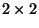Next: Area of a parallelogram Up: Applications of Determinant Previous: Introduction

## Definition of Determinant

Determinant is a function which as an input acceptsmatrix and out put is a real or a complex number that is called the determinant of the input matrix. One way to define determinant of anmatrixis the following formula:Where the terms are summed over all permutations, and the sign is + if the permutation is even, otherwise it is -.

There are easier ways to compute the determinant rather than using this formula. For example determinant of amatrixcan be find byand determinant of amatrixcan be find by writing the first two columns ofas columns 4 and 5 and then compute the sum of the products entries of the first three diagonals from left to right mines the sum of the products entries of the first three diagonals from right to left.There are other ways of computing the determinant of a given matrix, for example row operations can be used to reduce the matrix to a triangular matrix whose determinant is the product of the diagonal entries, another way is using cofactor expansion. In MATLAB you can use the command " det(A)" to compute the determinant of a given square matrix.

You may find more information about determinant in the following web sites:Next: Area of a parallelogram Up: Applications of Determinant Previous: Introduction
Ali A. Daddel 2000-09-15